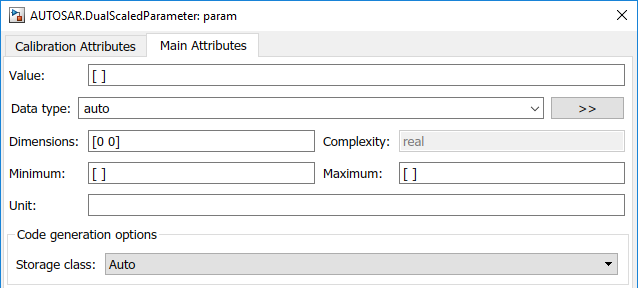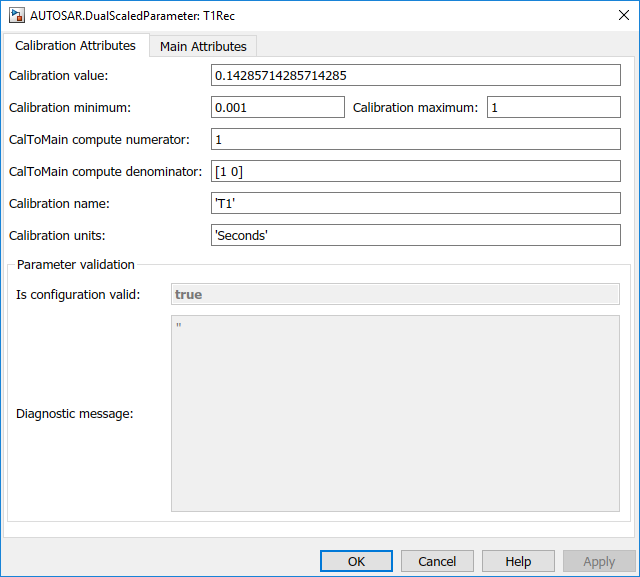# AUTOSAR.DualScaledParameter

Specify computation method, calibration value, data type, and other properties of AUTOSAR dual-scaled parameter

For run-time calibration of AUTOSAR data, R2018b and R2019a introduced graphical mapping of model elements to AUTOSAR component parameters and variables. In the Code Mappings editor, you select a Simulink® model-workspace parameter or internal signal, state, or data store. You map the selected element to an AUTOSAR component parameter or variable and modify its AUTOSAR calibration attributes. If you currently model AUTOSAR parameters or variables by using AUTOSAR parameter or signal objects in the base workspace, consider migrating to the Code Mappings editor workflow. For more information, see Map AUTOSAR Elements for Code Generation.

## Description

This class extends the `AUTOSAR.Parameter` class so that you can define an object that stores two scaled values of the same physical value. For example, for temperature measurement, you can store a Fahrenheit scale and a Celsius scale with conversion defined by a computation method that you provide. Given one scaled value, the `AUTOSAR.DualScaledParameter` can compute the other scaled value using the computation method.

A dual-scaled parameter has:

• A calibration value. The value that you prefer to use.

• A main value. The real-world value that Simulink uses.

• An internal stored integer value. The value that is used in the embedded code.

You can use `AUTOSAR.DualScaledParameter` objects in your model for both simulation and code generation. The parameter computes the internal value before code generation via the computation method. This offline computation results in leaner generated code.

If you provide the calibration value, the parameter computes the main value using the computation method. This method can be a first-order rational function.

`$y=\frac{ax+b}{cx+d}$`

• `x` is the calibration value.

• `y` is the main value.

• `a` and `b` are the coefficients of the CalToMain compute numerator.

• `c` and `d` are the coefficients of the CalToMain compute denominator.

If you provide the calibration minimum and maximum values, the parameter computes minimum and maximum values of the main value. Simulink performs range checking of parameter values. The software alerts you when the parameter object value lies outside a range that corresponds to its specified minimum and maximum values and data type.

## Creation

Create an `AUTOSAR.DualScaledParameter` object by using the `AUTOSAR.DualScaledParameter` function described below.

### Syntax

``DSParam = AUTOSAR.DualScaledParameter``

### Description

example

````DSParam = AUTOSAR.DualScaledParameter` returns an `AUTOSAR.DualScaledParameter` object with default property values.Open the workspace object to view and modify its properties.```

## Properties

The Main Attributes tab shows the properties inherited from the `AUTOSAR.Parameter` class. For more information, see `AUTOSAR.Parameter`.The Calibration Attributes tab shows calibration properties for the dual-scaled parameter.Calibration value

Calibration value of the parameter. The value that you prefer to use. The default value is `[]` (unspecified). Specify a finite, real, double value.

Before specifying Calibration value, you must specify CalToMain numerator and CalToMain denominator to define the computation method. The parameter uses the computation method and the calibration value to calculate the real-world value that Simulink uses.

Calibration minimum

Minimum value for the calibration parameter. The default value is `[]` (unspecified). Specify a finite, real, double scalar value.

Before specifying Calibration minimum, you must specify CalToMain numerator and CalToMain denominator to define the computation method. The parameter uses the computation method and the calibration minimum value to calculate the minimum or maximum value that Simulink uses. A first order rational function is strictly monotonic, either increasing or decreasing. If it is increasing, setting the calibration minimum sets the main minimum value. If it is decreasing, setting the calibration minimum sets the main maximum.

If the parameter value is less than the minimum value or if the minimum value is outside the range of the parameter data type, Simulink generates a warning. In these cases, when updating the diagram or starting a simulation, Simulink generates an error.

Calibration maximum

Maximum value for the calibration parameter can have. The default value is `[]` (unspecified). Specify a finite, real double scalar value.

Before specifying Calibration maximum, you must specify CalToMain numerator and CalToMain denominator to define the computation method. The parameter uses the computation method and the calibration maximum value to calculate the corresponding maximum or minimum value that Simulink uses. A first order rational function is strictly monotonic, either increasing or decreasing. If it is increasing, setting the calibration maximum sets the main maximum value. If it is decreasing, setting the calibration maximum sets the main minimum.

If the parameter value is less than the minimum value or if the minimum value is outside the range of the parameter data type, Simulink generates a warning. In these cases, when updating the diagram or starting a simulation, Simulink generates an error.

CalToMain compute numerator

Specify the numerator coefficients `a` and `b` of the first-order linear equation:

`$y=\frac{ax+b}{cx+d}$`

The default value is `[]` (unspecified). Specify finite, real double scalar values for `a` and `b`. For example, `[1 1]` or, for reciprocal scaling, `1`.

Once you have applied CalToMain compute numerator, you cannot change it.

CalToMain compute denominator

Specify the denominator coefficients `c` and `c` of the first-order linear equation:

`$y=\frac{ax+b}{cx+d}$`

The default value is `[]` (unspecified). Specify finite, real, double scalar values for `c` and `d`. For example, `[1 1]`.

Once you have applied CalToMain compute denominator, you cannot change it.

Calibration name

Specify the name of the calibration parameter. The default value is `''`. Specify a text value, for example, `'T1'`.

Calibration units

Specify the measurement units for this calibration value. This field is intended for use in documenting this parameter. The default value is `''`. Specify a text value, for example, `'Seconds'`.

Is configuration valid

Simulink indicates whether the configuration is valid. The default value is `true`. If Simulink detects an issue with the configuration, it sets this field to `false` and provides information in the Diagnostic message field. You cannot set this field.

Diagnostic message

If you specify invalid parameter settings, Simulink displays a message in this field. Use the diagnostic information to help you fix an invalid configuration issue. You cannot set this field.

This table shows command-line information for the calibration properties.

Name

Access

Description

`CalibrationValue`

`RW`

Calibration value of this parameter. See Calibration value.

`CalibrationMin`

`RW`

Calibration minimum value of this parameter. See Calibration minimum.

`CalibrationMax`

`RW`

Calibration maximum value of this parameter. See Calibration maximum.

`CalToMainCompuNumerator`

`RW`

Numerator coefficients of the computation method. See CalToMain compute numerator.

Once you have applied `CalToMainCompuNumerator`, you cannot change it.

`CalToMainCompuDenominator`

`RW`

Denominator coefficients of the computation method. See CalToMain compute denominator.

Once you have applied `CalToMainCompuDenominator`, you cannot change it.

`CalibrationName`

`RW`

Name of the calibration parameter. See Calibration name.

`CalibrationDocUnits`

`RW`

Measurement units for this calibration parameter's value. See Calibration units.

`IsConfigurationValid`

`RO`

Information about validity of configuration. See Is configuration valid.

`DiagnosticMessage`

`RO`

If the configuration is invalid, diagnostic information to help you fix the issue. See Diagnostic message.

## Examples

collapse all

Create an `AUTOSAR.DualScaledParameter` object that stores a value as both time and frequency.

`T1Rec = AUTOSAR.DualScaledParameter;`

Set the computation method.

```T1Rec.CalToMainCompuNumerator = ; T1Rec.CalToMainCompuDenominator = [1 0];```

This computation method specifies that the value used by Simulink is the reciprocal of the value that you want to use.

Set the value that you want to see.

`T1Rec.CalibrationValue = 1/7`
```T1Rec = DualScaledParameter with properties: CalibrationValue: 0.1429 CalibrationMin: [] CalibrationMax: [] CalToMainCompuNumerator: 1 CalToMainCompuDenominator: [1 0] CalibrationName: '' CalibrationDocUnits: '' IsConfigurationValid: 1 DiagnosticMessage: '' Value: 7 CoderInfo: [1×1 Simulink.CoderInfo] Description: '' DataType: 'auto' Min: [] Max: [] Unit: '' Complexity: 'real' Dimensions: [1 1]```

The `AUTOSAR.DualScaledParameter` calculates `T1Rec.Value` which is the value that Simulink uses. `T1Rec.CalibrationValue` is `1/7`, so `T1Rec.Value` is `7`.

Name this value and specify the units.

```T1Rec.CalibrationName = 'T1'; T1Rec.CalibrationDocUnits = 'Seconds';```

Set calibration minimum and maximum values.

```T1Rec.CalibrationMin = 0.001; T1Rec.CalibrationMax = 1;```

If you specify a value outside this allowable range, Simulink generates a warning.

Specify the units that Simulink uses.

`T1Rec.Unit = 'Hz';`

Open the AUTOSAR.DualScaledParameter dialog box.

`open T1Rec`The Calibration Attributes tab displays the calibration value and the computation method that you specified.

In the dialog box, click the Main Attributes tab.Create a dual-scaled parameter. Configure its storage class so that when you generate code, the exported ARXML code includes the dual-scaled parameter.

Create a dual-scaled parameter.

```T1Rec = AUTOSAR.DualScaledParameter; T1Rec.CalToMainCompuNumerator = ; T1Rec.CalToMainCompuDenominator = [1 0]; T1Rec.CalibrationValue = 1/7; T1Rec.CalibrationName = 'T1'; T1Rec.CalibrationDocUnits = 'Seconds'; T1Rec.CalibrationMin = 0.001; T1Rec.CalibrationMax = 1```
```T1Rec = DualScaledParameter with properties: CalibrationValue: 0.1429 CalibrationMin: 1.0000e-03 CalibrationMax: 1 CalToMainCompuNumerator: 1 CalToMainCompuDenominator: [1 0] CalibrationName: 'T1' CalibrationDocUnits: 'Seconds' IsConfigurationValid: 1 DiagnosticMessage: '' Value: 7 CoderInfo: [1×1 Simulink.CoderInfo] Description: '' DataType: 'auto' Min: 1 Max: 1000 Unit: '' Complexity: 'real' Dimensions: [1 1]```

Set the storage class of the parameter so that the generated ARXML code includes the parameter.

```T1Rec.CoderInfo.StorageClass = 'Custom'; T1Rec.CoderInfo.CustomStorageClass = 'InternalCalPrm';```

You can now use the parameter in a Simulink model. If you configure the model for AUTOSAR, when you generate code for the model, the code generator produces ARXML code that contains information about the dual-scaled parameter.